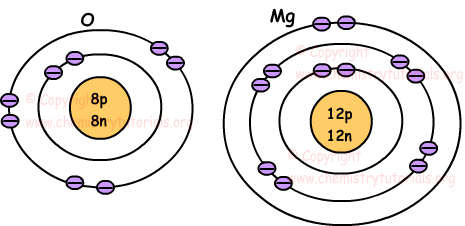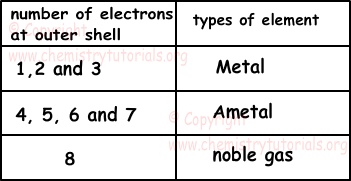## Electron Configuration with Examples

Electron Configuration with Examples

Electrons are not placed at fixed positions in atoms, but we can predict approximate positions of them. These positions are called energy levels or shells of atoms.

• Lowest energy level is 1 and it is denoted with integer n=1, 2, 3, 4, 5, 6... or letters starting from K, L, N to Q.  An atom can have maximum 7 energy levels and electrons can change their levels according to their energies.
• Each energy level has different number of electrons. For example, we can find number of electrons in four energy level with following formula; 2n2.

1st energy level has;

2n2=2.12=2 electrons

2nd energy level has;

2n2=2.22=8 electrons

3rd energy level has;

2n2=2.32=18 electrons

• Electrons are located energy levels starting from the first energy levels. If one of the energy level is full, then electrons are placed following energy level.

Following pictures show location of electrons of atoms O and Mg.Number of electrons at the outer shell of atom gives us following classification.Electron configuration of atom shows, shells, sub shells and number of electrons in sub shells. We examine electron configuration with following examples.

Example: Helium 2

1s2

Where;

1 is the principal quantum number or energy level (shell)

s is the sub-level or sub shell (Capacity of s sub shell is 2 electron)

2 shows the number of electrons in the s sub shell

Example: Chlorine 17

1s22s22p63s23p5

Coefficients 1, 2, 2, 3, and 3 are energy levels of Cl. As you can see "p" sub shell can have maximum 6 electrons.

Superscripts 2, 2, 6, 2 and 5 are electrons in the sub shells "s" and "p".

Example: Bromine 35

1s22s22p63s23p64s23d104p5

As you can see "d" sub shell can have maximum 10 electrons.

Example: Tantalum 73

1s22s22p63s23p64s23d104p65s24d105p66s24f145d3

As you can see "f" sub shell can have maximum 14 electrons.

Atomic Structure Exams and  Problem Solutions

Related